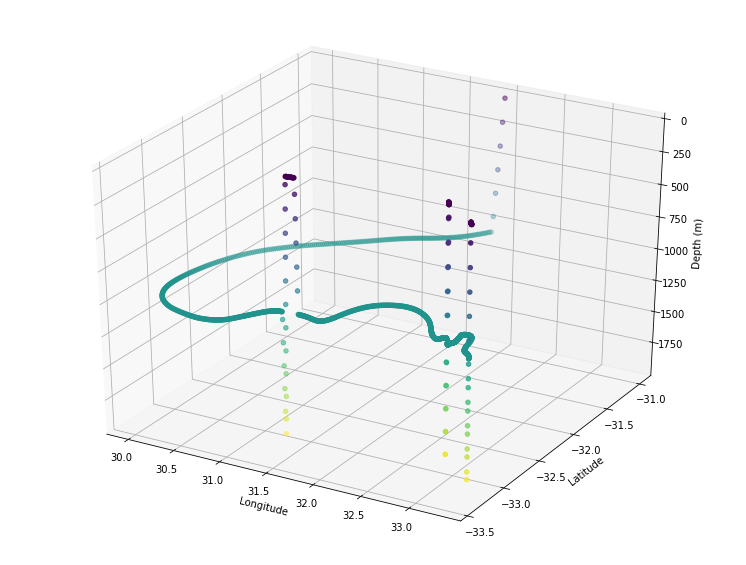## Tutorial on how to simulate an Argo float in Parcels¶

This tutorial shows how simple it is to construct a Kernel in Parcels that mimics the vertical movement of Argo floats.

In :
# Define the new Kernel that mimics Argo vertical movement
def ArgoVerticalMovement(particle, fieldset, time):
driftdepth = 1000  # maximum depth in m
maxdepth = 2000  # maximum depth in m
vertical_speed = 0.10  # sink and rise speed in m/s
cycletime = 10 * 86400  # total time of cycle in seconds
drifttime = 9 * 86400  # time of deep drift in seconds

if particle.cycle_phase == 0:
# Phase 0: Sinking with vertical_speed until depth is driftdepth
particle.depth += vertical_speed * particle.dt
if particle.depth >= driftdepth:
particle.cycle_phase = 1

elif particle.cycle_phase == 1:
# Phase 1: Drifting at depth for drifttime seconds
particle.drift_age += particle.dt
if particle.drift_age >= drifttime:
particle.drift_age = 0  # reset drift_age for next cycle
particle.cycle_phase = 2

elif particle.cycle_phase == 2:
# Phase 2: Sinking further to maxdepth
particle.depth += vertical_speed * particle.dt
if particle.depth >= maxdepth:
particle.cycle_phase = 3

elif particle.cycle_phase == 3:
# Phase 3: Rising with vertical_speed until at surface
particle.depth -= vertical_speed * particle.dt
#particle.temp = fieldset.temp[time, particle.depth, particle.lat, particle.lon]  # if fieldset has temperature
if particle.depth <= fieldset.mindepth:
particle.depth = fieldset.mindepth
#particle.temp = 0./0.  # reset temperature to NaN at end of sampling cycle
particle.cycle_phase = 4

elif particle.cycle_phase == 4:
# Phase 4: Transmitting at surface until cycletime is reached
if particle.cycle_age > cycletime:
particle.cycle_phase = 0
particle.cycle_age = 0

if particle.state == ErrorCode.Evaluate:
particle.cycle_age += particle.dt  # update cycle_age


And then we can run Parcels with this 'custom kernel'.

Note that below we use the two-dimensional velocity fields of GlobCurrent, as these are provided as example_data with Parcels.

We therefore assume that the horizontal velocities are the same throughout the entire water column. However, the ArgoVerticalMovement kernel will work on any FieldSet, including from full three-dimensional hydrodynamic data.

If the hydrodynamic data also has a Temperature Field, then uncommenting the lines about temperature will also simulate the sampling of temperature.

In :
from parcels import FieldSet, ParticleSet, JITParticle, AdvectionRK4, ErrorCode, Variable
from datetime import timedelta
import numpy as np

# Load the GlobCurrent data in the Agulhas region from the example_data
filenames = {'U': "GlobCurrent_example_data/20*.nc",
'V': "GlobCurrent_example_data/20*.nc"}
variables = {'U': 'eastward_eulerian_current_velocity',
'V': 'northward_eulerian_current_velocity'}
dimensions = {'lat': 'lat', 'lon': 'lon', 'time': 'time'}
fieldset = FieldSet.from_netcdf(filenames, variables, dimensions)
fieldset.mindepth = fieldset.U.depth  # uppermost layer in the hydrodynamic data

# Define a new Particle type including extra Variables
class ArgoParticle(JITParticle):
# Phase of cycle: init_descend=0, drift=1, profile_descend=2, profile_ascend=3, transmit=4
cycle_phase = Variable('cycle_phase', dtype=np.int32, initial=0.)
cycle_age = Variable('cycle_age', dtype=np.float32, initial=0.)
drift_age = Variable('drift_age', dtype=np.float32, initial=0.)
#temp = Variable('temp', dtype=np.float32, initial=np.nan)  # if fieldset has temperature

# Initiate one Argo float in the Agulhas Current
pset = ParticleSet(fieldset=fieldset, pclass=ArgoParticle, lon=, lat=[-31], depth=)

# combine Argo vertical movement kernel with built-in Advection kernel
kernels = ArgoVerticalMovement + pset.Kernel(AdvectionRK4)

# Create a ParticleFile object to store the output
output_file = pset.ParticleFile(name="argo_float", outputdt=timedelta(minutes=30))

# Now execute the kernels for 30 days, saving data every 30 minutes
pset.execute(kernels, runtime=timedelta(days=30), dt=timedelta(minutes=5), output_file=output_file)

WARNING: Casting lon data to np.float32
WARNING: Casting lat data to np.float32
WARNING: Casting depth data to np.float32
INFO: Compiled ArgoParticleArgoVerticalMovementAdvectionRK4 ==> /var/folders/r2/8593q8z93kd7t4j9kbb_f7p00000gr/T/parcels-504/9b8a821c179c51426159d7b7efb2e070.so
100% (2592000.0 of 2592000.0) |##########| Elapsed Time: 0:00:10 Time:  0:00:10


Now we can plot the trajectory of the Argo float with some simple calls to netCDF4 and matplotlib

In :
%matplotlib inline
import netCDF4
from mpl_toolkits.mplot3d import Axes3D
import matplotlib.pyplot as plt

output_file.export()  # export the trajectory data to a netcdf file

nc = netCDF4.Dataset("argo_float.nc")
x = nc.variables["lon"][:].squeeze()
y = nc.variables["lat"][:].squeeze()
z = nc.variables["z"][:].squeeze()
nc.close()

fig = plt.figure(figsize=(13,10))
ax = plt.axes(projection='3d')
cb = ax.scatter(x, y, z, c=z, s=20, marker="o")
ax.set_xlabel("Longitude")
ax.set_ylabel("Latitude")
ax.set_zlabel("Depth (m)")
ax.set_zlim(np.max(z),0)
plt.show()In [ ]: